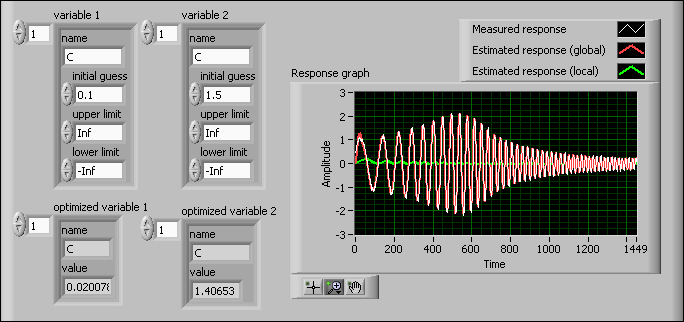# Setting Parameter Constraints with an Initial Guess (System Identification Toolkit)

If you have information about a certain parameter and can estimate a value for that parameter, you can refine estimation by using that value as an initial guess.

The SI Estimate Partially Known State-Space Model VI and the SI Estimate Partially Known Continuous Transfer Function Model VI perform optimization using the initial guess you provide. These two VIs then use the upper and lower limit settings you specify as boundary constraints during the optimization process.

The initial guess you provide greatly affects the performance of any optimization technique. Whether an optimization process reaches a global optimum depends on the initial guess. With some initial guesses, optimization processes might locate only a local optimum, which is the smallest difference between the estimated output and the real output within a certain smaller range rather than in the whole range of interest. Therefore, to decrease the risk of locating a local optimum instead of the global optimum, try different initial guesses. The following figure shows an example of different estimations resulting from different initial guesses and illustrates the importance of setting different initial guesses to find the global optimum.As the previous figure shows, if you set C to an initial guess of 0.1, you obtain an optimized value of 0.02. You can see the Estimated response (global) plot and the Measured response plot match in the Response graph. This response from the estimated model is close to the real-world model response. However, if you set the initial guess of C to 1.5, you get an optimized value of 1.41. The Estimated response (local) plot does not match the Measured response plot in the Response graph. Thus, with this initial guess, the estimated model response does not represent the real-world model response accurately.